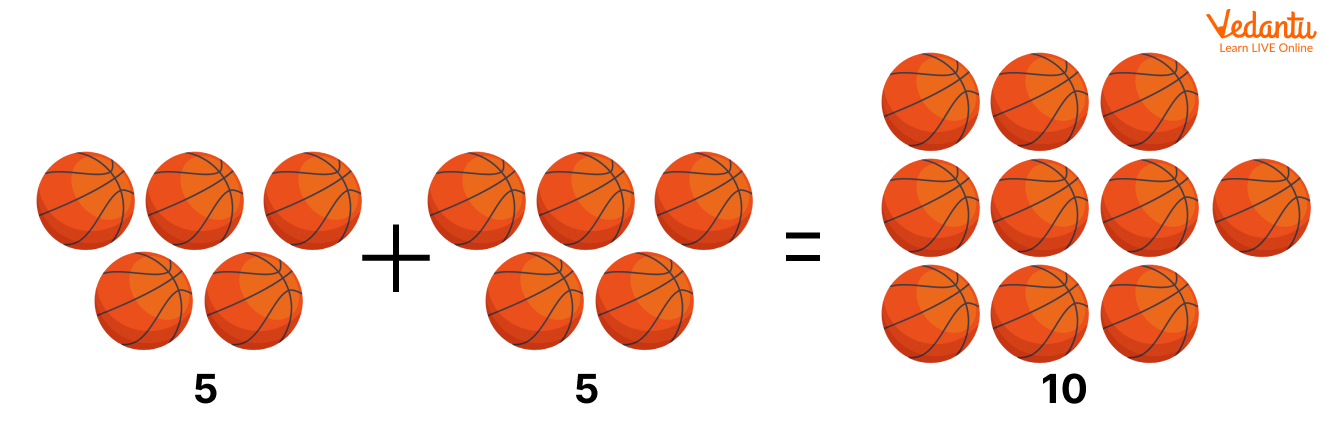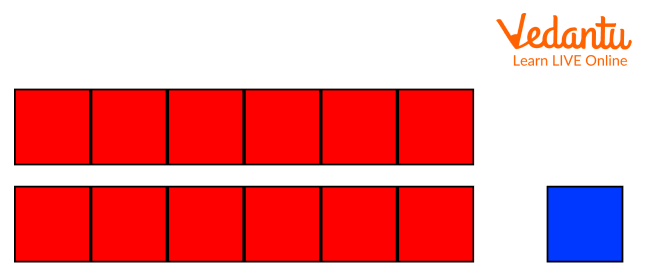Courses
Courses for Kids
Free study material
Free LIVE classes
More

# Double Plus One Facts: A Simple Method To Add Two Consecutive Numbers## What is Double Fact Strategy in Math?

Let us first understand the meaning of Double fact before coming into the double plus one fact. Once you understand the double fact strategy, you can use this to understand double plus one fact in a better way.

When we add any two same numbers, it implies a double fact.

For example:

3 + 3 and 5 + 5 are double facts.

Look at the image below to understand the double fact in a more effective way.Double Plus Fact

Here, 5 + 5 = 10 is a double fact because we are adding the same number “5”. Now, we can use this strategy to understand double plus one fact.

### What Is Double Plus One Fact?

Double Plus One Fact is a strategy used to add two consecutive numbers (numbers that are next to each other). In this strategy, you simply add a smaller number to itself or double it and add 1 to get the final result.

Let us understand double plus one facts with example:

In the example below, two consecutive numbers i.e. 6 and 7 have been added using the double plus strategy.

6 + 76 + 7 = ? 6 + 7 = 6 + 6 + 1

↙ ↘ 13 = 12 + 1

6 1 13 = 13

In the above example, we have splitted 7 as (6 + 1 = 7)

Below are a few more examples of double plus one strategy.

Example 1:+2 stars 3 stars

As we know 2 + 2 = 4 so 2 + 3 = 5

According to double plus one fact strategy, it will be written as:

2 + 3 = 2 + 2 + 1

5 = 5

Example 2:+5 Apples 6 Apples

As we know 5 + 5 = 10 so 5 + 6 = 11

According to double plus one fact strategy, it will be written as:

5 + 6 = 5 + 5 + 1

11 = 11

Do You Know?

• Double fact states that a double of 3 is 6 because 3 + 3 = 6.

• To use double fact in Math, simply add the number to itself. For example, 4 + 4 = 8.

• Near doubles or double plus one fact is a single digit away from double facts. For example, 4 + 4 is a double fact and 4 + 5 is a near double or double plus one fact.

### Conclusion:

In short, double plus one fact states that ‘double first’ and then just “count on one”. This enables you to answer the questions of how adjacent or consecutive numbers are added. For example, to add 7 + 8, double 7 and add 1. Accordingly, 7 + 7 + 1 = 15.

Last updated date: 20th Sep 2023
Total views: 137.1k
Views today: 3.37k

## FAQs on Double Plus One Facts: A Simple Method To Add Two Consecutive Numbers

1. What do near doubles mean in Math?

Near doubles are those addends that are almost close to double facts. For example, 6 + 7 is very close to 6 + 6.  You can easily recall the double fact for 6 + 6 = 12, and by adding 1 more. This can be speedily estimated as 6 + 7 = 13.

2. What is a double plus one fact strategy?

It is a strategy used to add two consecutive numbers or adjacent numbers together. In this strategy, we simply add the smaller number to itself or double it and then add 1 to get the final answer. For example, consecutive numbers 3 and 4 using the double plus one fact strategy can be added as 3 + 3 + 1 = 7.

3. What does Doubles mean in Math?

In Math, double means to simply add the same number itself. For example, a double of 5 is 5 + 5 = 10. It is quite easy to remember the numbers we get by doubling one-digit numbers.